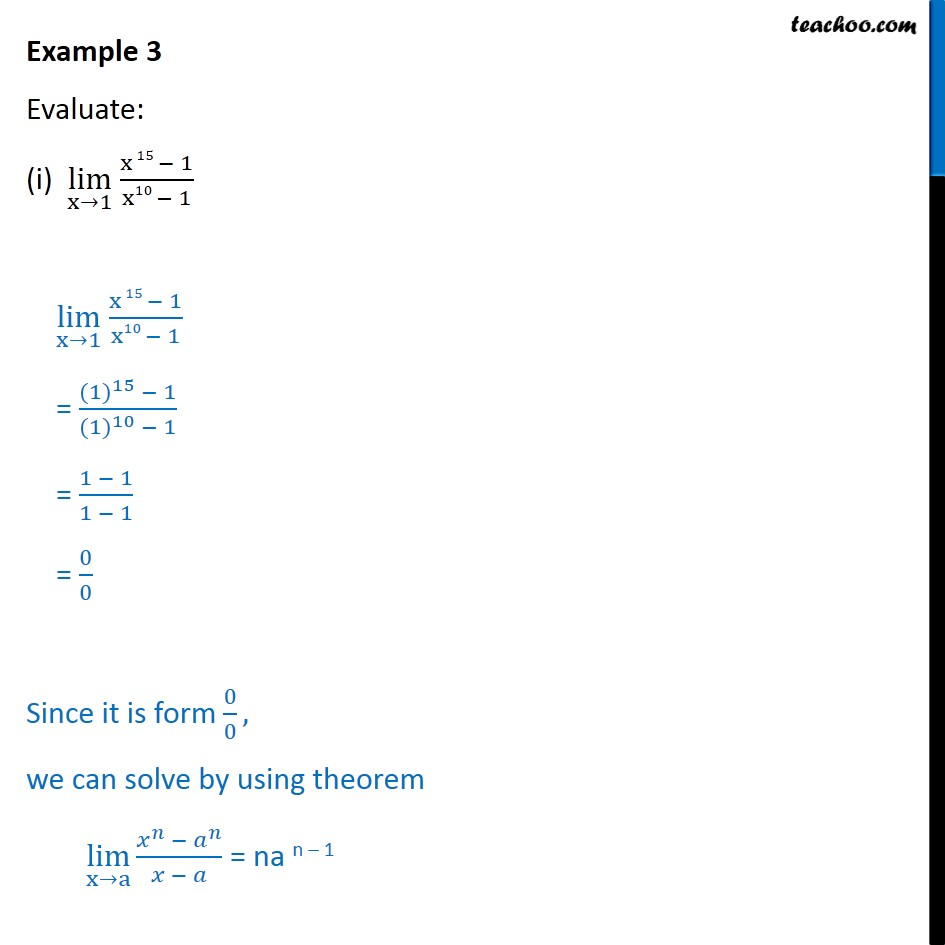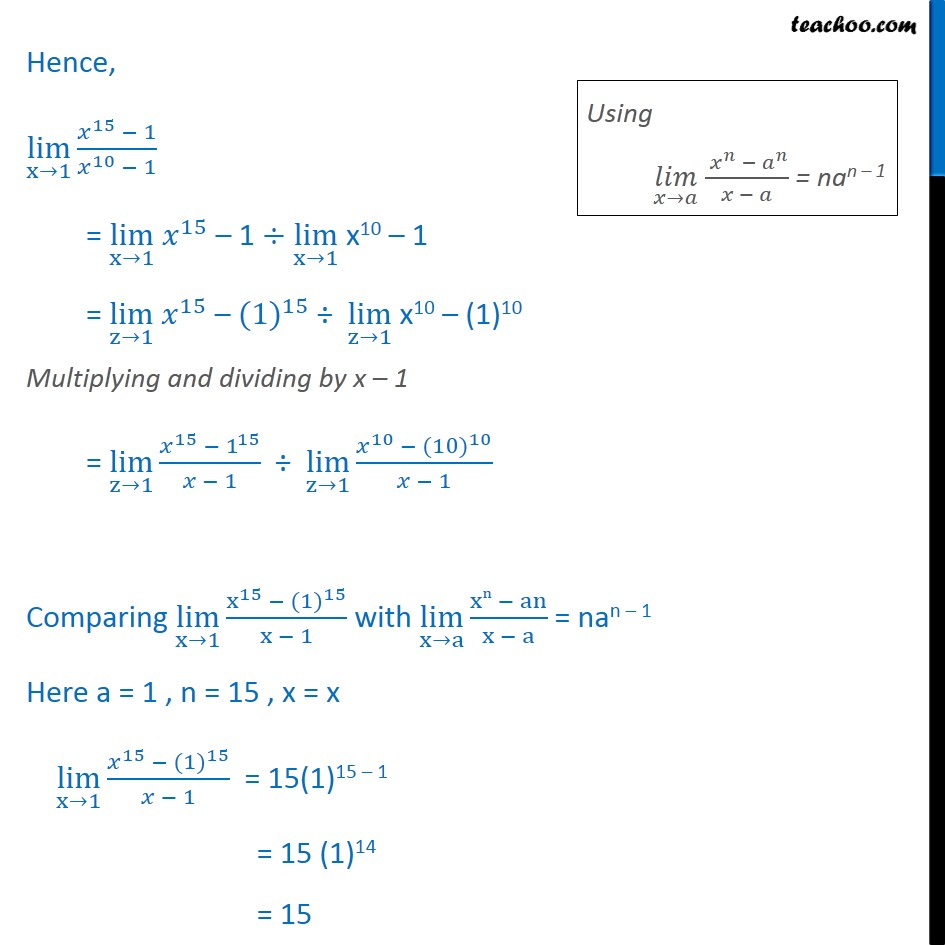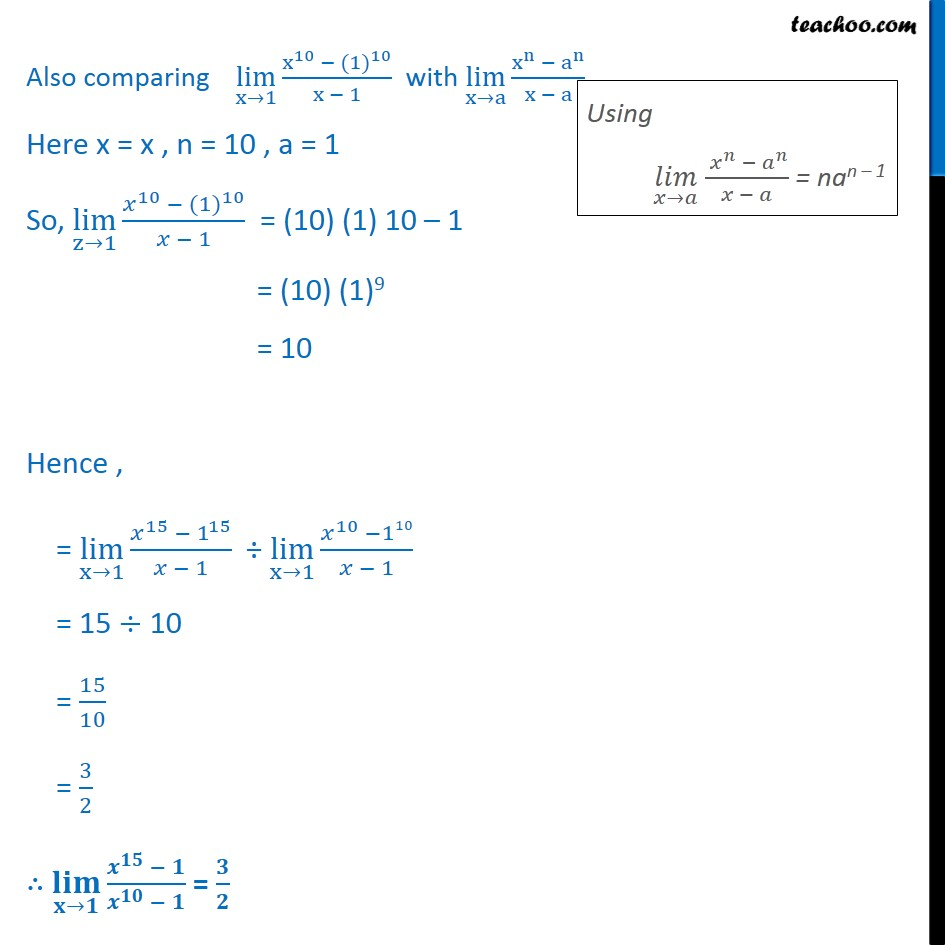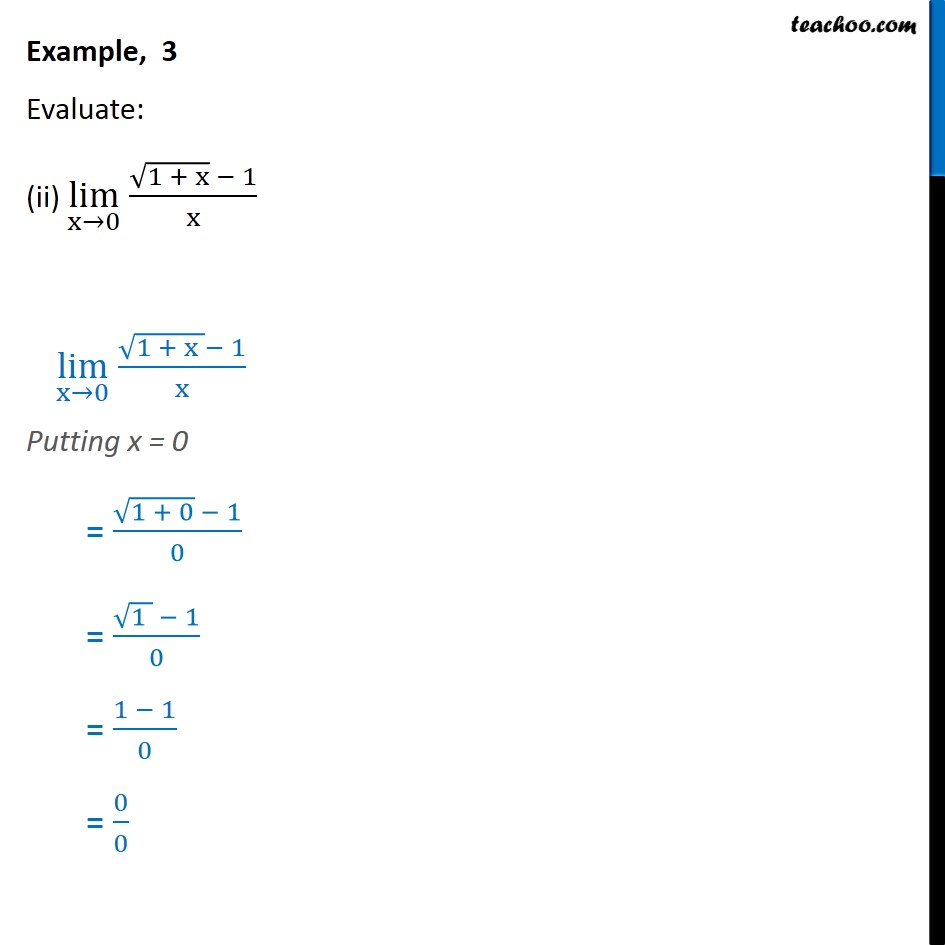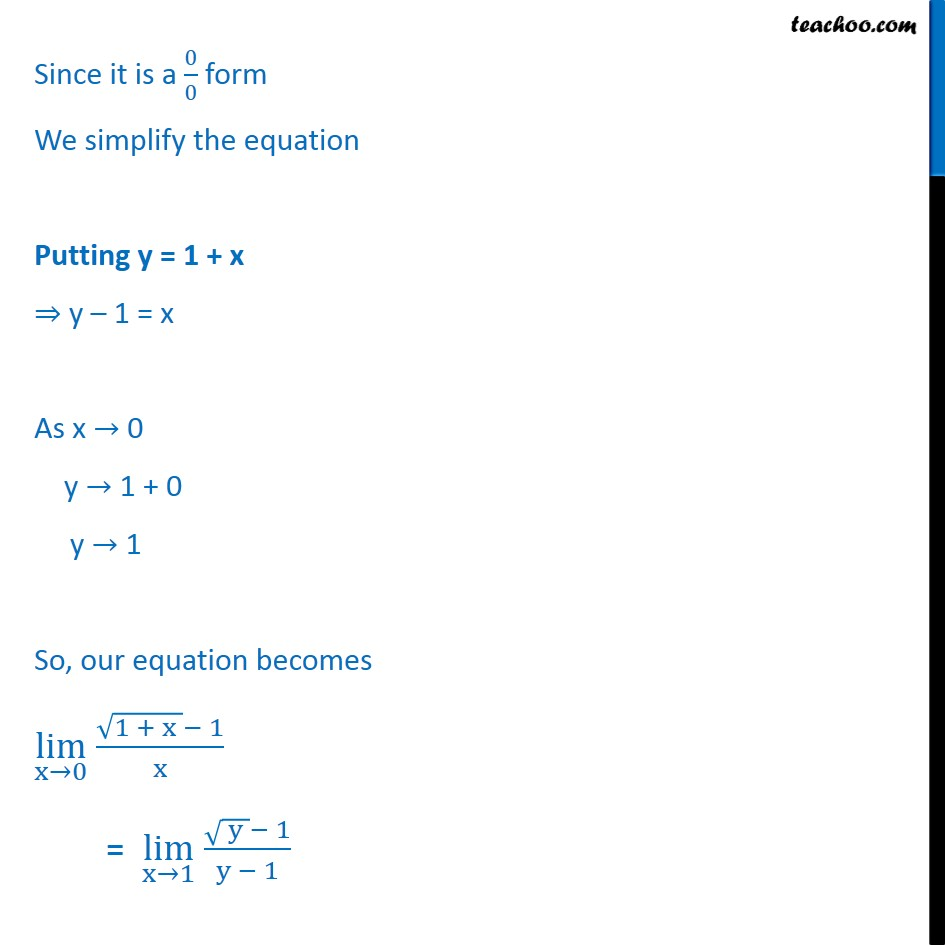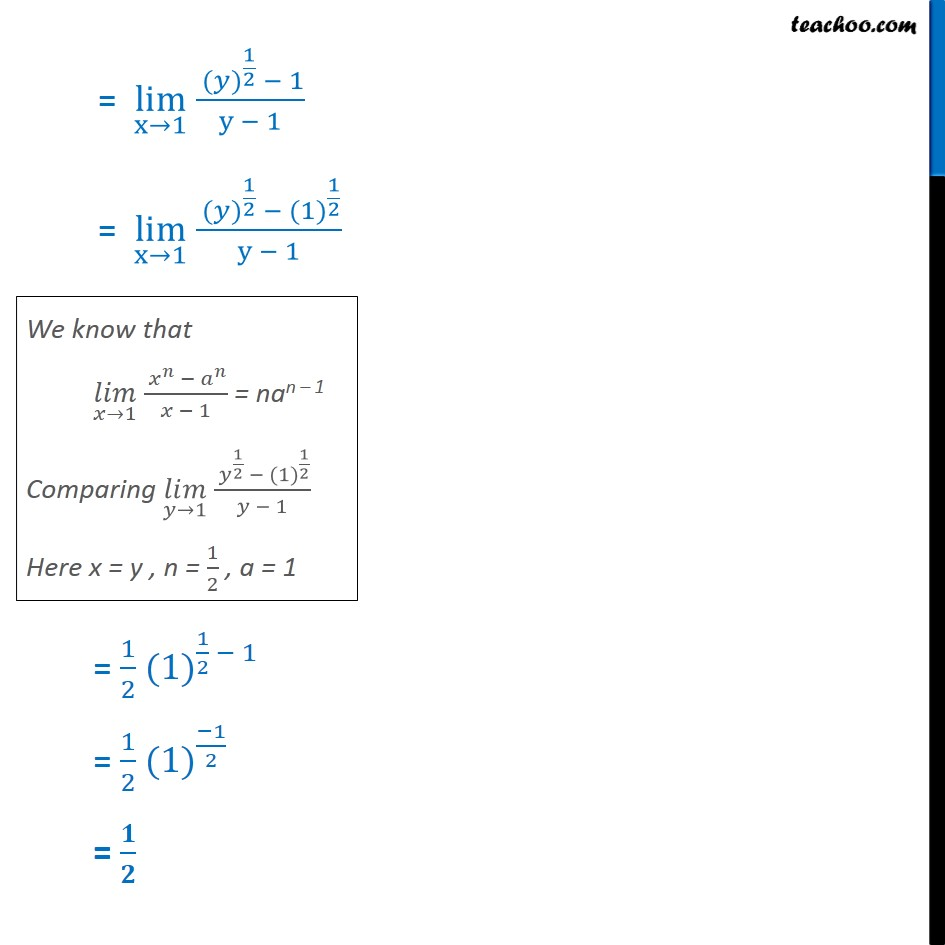1. Chapter 13 Class 11 Limits and Derivatives
2. Serial order wise
3. Examples

Transcript

Example 3 Evaluate: (i) lim (x 1) (x 15 1)/(x10 1) lim (x 1) (x 15 1)/(x10 1) = ( (1) ^15 1)/( (1) ^10 1) = (1 1)/(1 1) = 0/0 Since it is form 0/0, we can solve by using theorem lim (x a) ( ^ ^ )/( ) = na n 1 Hence, lim (x 1) ( ^15 1)/( ^10 1) = lim (x 1) ^15 1 lim (x 1) x10 1 = lim (z 1) ^15 (1) ^15 lim (z 1) x10 (1)10 Multiplying and dividing by x 1 = lim (z 1) ( ^15 1^15)/( 1) lim (z 1) ( ^10 (10) ^10)/( 1) Comparing lim (x 1) (x^15 (1) ^15)/(x 1) with lim (x a) (xn an)/(x a) = nan 1 Here a = 1 , n = 15 , x = x lim (x 1) ( ^15 (10) ^15)/( 1) = 15(1)15 1 = 15 (1)14 = 15 Also comparing lim (x 1) (x^10 (1) ^10)/(x 1) with lim (x a) (x^n a^n)/(x a) Here x = x , n = 10 , a = 1 So, lim (z 1) ( ^10 (1) ^10)/( 1) = (10) (1) 10 1 = (10) (1)9 = 10 Hence , = lim (x 1) ( ^15 1^15)/( 1) lim (x 1) ( ^10 110)/( 1) = 15 10 = 15/10 = 3/2 ( ) ( ) ( ^ )/( ^ ) = / Example, 3 Evaluate: (ii) lim (x 0) ( (1 + x) 1)/x lim (x 0) ( (1 + x ) 1)/x Putting x = 0 = ( (1 + 0) 1)/0 = ( (1 ) 1)/0 = (1 1)/0 = 0/0 Since it is a 0/0 form We simplify the equation Putting y = 1 + x y 1 = x As x 0 y 1 + 0 y 1 So, our equation becomes lim (x 0) ( (1 + x ) 1)/x = lim (x 1) ( ( y ) 1)/(y 1) = lim (x 1) ( ( ) ^(1/2) 1)/(y 1) = lim (x 1) ( ( ) ^(1/2) (1) ^(1/2))/(y 1) = 1/2 (1) ^(1/2 1) = 1/2 (1) ^(1/2) = /

Examples